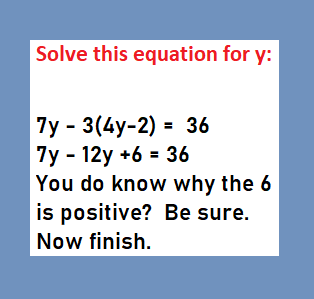# Solve equation that has parenthesis

Posted on: March 22, 2020 Posted by: Susan Johnsey Comments: 15x – 10 = 3x – 10 – (x – 2 )

First you must multiply the x – 2 by -1 before you begin  your steps of solving. The – (x – 2 ) is the same as – 1(x – 2 ) . So 5x – 10 = ????

I want the x-terms on the left side of the “=” and the number terms on the right side.  But first simplify the right side. You will make fewer mistakes.  5x – 10 = 3x – 10 – x +2. You do know why the 2 is positive?

Simplifies to 5x-10 = 2x – 8.

NOW begin solving. I will begin solving:  Subtract 2x from both sides.

This is how we “move the x-term” to the left side of the equation.

3x – 10 = – 8     Now ADD 10 to both sides.  This is how we move the “-10 term” to the right side of the equation.
Did you notice that the 10 is negative, and that it is on the left side of the equation?

3x – 10 + 10 =-8 + 10      This becomes: 3x =2

How do we finish with 1x instead
of 3x?  Divide by 3 or
multiply by 1/3 .

Thus 3x =2    becomes   3x/3 = 2/3 ,  I divided by 3 (or  multiplied by 1/3).    So we know x= 2/3.

YES,  divide by 3 or multiply by 1/3 are the same!!

### 1 people reacted on this

1.Susan Johnsey says:

Thanks